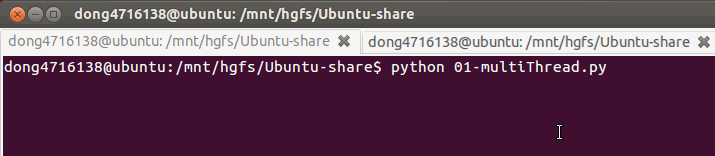## 1. 线程执行代码的封装

``````    #coding=utf-8
import time

def run(self):
for i in range(3):
time.sleep(1)
msg = "I'm "+self.name+' @ '+str(i) #name属性中保存的是当前线程的名字
print(msg)

if __name__ == '__main__':
t.start()
``````## 2. 线程的执行顺序

``````#coding=utf-8
import time

def run(self):
for i in range(3):
time.sleep(1)
msg = "I'm "+self.name+' @ '+str(i)
print(msg)
def test():
for i in range(5):
t.start()
if __name__ == '__main__':
test()
``````

``````I'm Thread-1 @ 0
``````

## 3. 总结

1. 每个线程一定会有一个名字，尽管上面的例子中没有指定线程对象的name，但是python会自动为线程指定一个名字。
2. 当线程的run()方法结束时该线程完成。
3. 无法控制线程调度程序，但可以通过别的方式来影响线程调度的方式。
4. 线程的几种状态